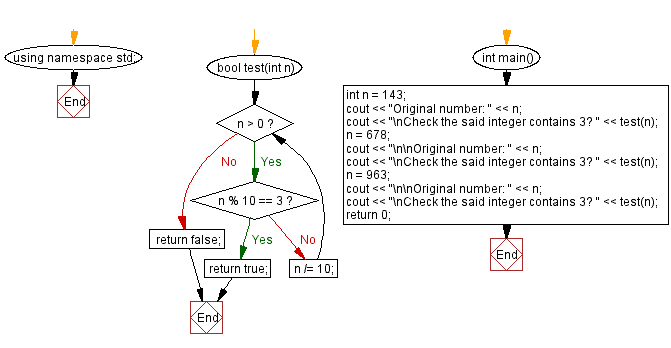﻿ CPP - Check whether an integer contains 3

# C++ Exercises: Test a positive integer and return true if it contains the number 2

## C++ Basic Algorithm: Exercise-122 with Solution

Write a C++ program to check a positive integer and return true if it contains the number 3. Otherwise return false.

Test Data:
(143) -> 1
(678) -> 0
(963) -> 1

Sample Solution:

C++ Code :

``````#include <iostream>

using namespace std;

bool test(int n)
{
while (n > 0)
{
if (n % 10 == 3) return true;
n /= 10;
}
return false;
}

int main() {

int n = 143;
cout << "Original number: " << n;
cout << "\nCheck the said integer contains 3? " << test(n);
n = 678;
cout << "\n\nOriginal number: " << n;
cout << "\nCheck the said integer contains 3? " << test(n);
n = 963;
cout << "\n\nOriginal number: " << n;
cout << "\nCheck the said integer contains 3? " << test(n);
return 0;
}

``````

Sample Output:

```Original number: 143
Check the said integer contains 3? 1

Original number: 678
Check the said integer contains 3? 0

Original number: 963
Check the said integer contains 3? 1
```

Flowchart:C++ Code Editor:

What is the difficulty level of this exercise?

﻿

## C++ Programming: Tips of the Day

What is the usefulness of `enable_shared_from_this?

It enables you to get a valid shared_ptr instance to this, when all you have is this. Without it, you would have no way of getting a shared_ptr to this, unless you already had one as a member.

```class Y: public enable_shared_from_this
{
public:

shared_ptr f()
{
return shared_from_this();
}
}

int main()
{
shared_ptr p(new Y);
shared_ptr q = p->f();
assert(p == q);
assert(!(p < q || q < p)); // p and q must share ownership
}
```

The method f() returns a valid shared_ptr, even though it had no member instance. Note that you cannot simply do this:

```class Y: public enable_shared_from_this
{
public:

shared_ptr f()
{
return shared_ptr(this);
}
}
```

The shared pointer that this returned will have a different reference count from the "proper" one, and one of them will end up losing and holding a dangling reference when the object is deleted.

Ref : https://bit.ly/3pwVzzz